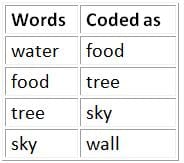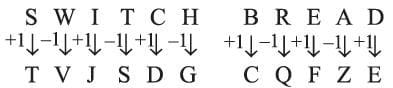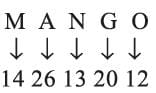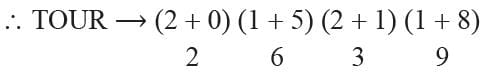Olympiad Test: Coding and Decoding

# Olympiad Test: Coding and Decoding

Test Description

## 15 Questions MCQ Test Science Olympiad Class 9 | Olympiad Test: Coding and Decoding

Olympiad Test: Coding and Decoding for Class 9 2022 is part of Science Olympiad Class 9 preparation. The Olympiad Test: Coding and Decoding questions and answers have been prepared according to the Class 9 exam syllabus.The Olympiad Test: Coding and Decoding MCQs are made for Class 9 2022 Exam. Find important definitions, questions, notes, meanings, examples, exercises, MCQs and online tests for Olympiad Test: Coding and Decoding below.
Solutions of Olympiad Test: Coding and Decoding questions in English are available as part of our Science Olympiad Class 9 for Class 9 & Olympiad Test: Coding and Decoding solutions in Hindi for Science Olympiad Class 9 course. Download more important topics, notes, lectures and mock test series for Class 9 Exam by signing up for free. Attempt Olympiad Test: Coding and Decoding | 15 questions in 15 minutes | Mock test for Class 9 preparation | Free important questions MCQ to study Science Olympiad Class 9 for Class 9 Exam | Download free PDF with solutions
 1 Crore+ students have signed up on EduRev. Have you?
Olympiad Test: Coding and Decoding - Question 1

### In a certain code ZIP = 198, VIP = 222 then ZAP will be equal to

Detailed Solution for Olympiad Test: Coding and Decoding - Question 1

Taking Z = 2, Y = 3, …… N = 14,
B = 26, A = 27
∴ ZIP = (2 + 19 + 12) × 6 = 33 × 6 = 198
and VIP = (V + I + P) × 6 = (6 + 19 + 12) × 6
= 37 × 6 = 222
Hence ZAP = (Z + A + P) × 6
= (2 + 27 + 12) × 6
= 41 × 6 = 246

Olympiad Test: Coding and Decoding - Question 2

### In a certain code 134 means ‘good and tasty’, 478 means ‘see good pictures’ 728 means ‘pictures are faint. Which of the following digit stands for pictures?

Detailed Solution for Olympiad Test: Coding and Decoding - Question 2

Given 134 → good and tasty
478 → see good pictures
728 → pictures are faint
Hence, 4 → Good and 8 → Pictures.

Olympiad Test: Coding and Decoding - Question 3

### If water is called food, food is called tree, the tree is called sky, the sky is called wall, then on which of the following grows a fruit?

Detailed Solution for Olympiad Test: Coding and Decoding - Question 3

The logic follows here is:As the fruits grow on a tree and tree is coded as the sky.
Hence, the correct answer is "Sky".

Olympiad Test: Coding and Decoding - Question 4

If the paper is called ‘wood’, wood is called straw, ‘straw is called grass, ‘grass is called rubber,’ rubber is called cloth then what is the furniture made up of?

Detailed Solution for Olympiad Test: Coding and Decoding - Question 4

Furniture is made up of wood and wood is straw (here).

Olympiad Test: Coding and Decoding - Question 5

If DRIVER = 12, CAR = 6, ACCIDENT = 16 then PEDESTRIAN = ?

Detailed Solution for Olympiad Test: Coding and Decoding - Question 5

Here DRIVER (6 × 2) = 12
CAR (3 × 2) = 6
ACCIDENT (8 × 2) = 16
∴ PEDESTRIAN = 10 × 2 = 20

Olympiad Test: Coding and Decoding - Question 6

In a certain code, SWITCH is coded as TVJSDG, which word would be written as CQFZE?

Detailed Solution for Olympiad Test: Coding and Decoding - Question 6

HereOlympiad Test: Coding and Decoding - Question 7

If MOBILITY is coded as 46293927 then EXAMINATION is coded as?

Detailed Solution for Olympiad Test: Coding and Decoding - Question 7

Here
M = 1 3 = 1 + 3 = 4; O = 15 + 1 + 5 = 6;
B = 2 , I = 9 , L =  12 = 1 + 2 = 3;
T = 20 = 2 + 0 = 2 Y = 25 + 2 + 5 = 7;
X = 2 4  = 2 + 4 = 6; N = 14 = 1 + 4 = 5
∴ EXAMINATION → 56149512965

Olympiad Test: Coding and Decoding - Question 8

If 2 = 5, 4 = 18, 6 = 39 then what is value of 10?

Detailed Solution for Olympiad Test: Coding and Decoding - Question 8

Here 2 = 22 + 1 = 5; 4 = 42 + 2 = 18;
6 =  62 + 3 = 39; 8 = 82 + 4 = 68;
10 = 102 + 5 = 105.

Olympiad Test: Coding and Decoding - Question 9

If the eye is called hand, the hand is called mouth, the mouth is called ear, the ear is called a nose, and the nose is called tongue then which of the following is used for finding a smell?

Detailed Solution for Olympiad Test: Coding and Decoding - Question 9

We can smell with nose. Here, nose is called tongue
Nose → Tongue.

Olympiad Test: Coding and Decoding - Question 10

In a code A = 26, C = 24, T = 7, X = 3 then what is the code for MANGO?

Detailed Solution for Olympiad Test: Coding and Decoding - Question 10

Here,Olympiad Test: Coding and Decoding - Question 11

If J = 1, L = 3, Z = 8, T = 2 then what is the code for TOUR?

Detailed Solution for Olympiad Test: Coding and Decoding - Question 11

J = 1 = 1 + 0
L = 3  = 1 + 2
Z = 8  = 2 + 6
T = 2  = 2 + 0Olympiad Test: Coding and Decoding - Question 12

If E = 5, I = 9, Y = 25, U = 21 then what is the code for TRAIN?

Detailed Solution for Olympiad Test: Coding and Decoding - Question 12

Here, E = 5, I = 9, Y = 25, U = 21
∴ TRAIN ⟶ 20181914

Olympiad Test: Coding and Decoding - Question 13

In certain code ON = 29; RAT = 39 the what is the code for COW?

Detailed Solution for Olympiad Test: Coding and Decoding - Question 13

Here ON = 29 = 15 + 14
and RAT = 39 = 18 + 1 + 20
∴ COW =  3 + 15 + 23 = 41

Olympiad Test: Coding and Decoding - Question 14

In a code language AT is coded as 401; CAT is coded as 410. What is the code for MAT?

Detailed Solution for Olympiad Test: Coding and Decoding - Question 14

AT → 401 ⇒12 + 202 = 1 + 400 = 401
CAT → 410 = 32 + 12 + 202 = 9 + 1 + 400 = 410
∴ MAT → 132 + 12 + 20
= 169 + 1 + 400 = 570

Olympiad Test: Coding and Decoding - Question 15

If Cat is called dog, dog is called snake, snake is called Lion. Lion is called Deer. Then which is called king of the forest?

Detailed Solution for Olympiad Test: Coding and Decoding - Question 15

Here Lion → Deer
Lion is called king of the forest.

## Science Olympiad Class 9

17 videos|6 docs|55 tests
 Use Code STAYHOME200 and get INR 200 additional OFF Use Coupon Code
Information about Olympiad Test: Coding and Decoding Page
In this test you can find the Exam questions for Olympiad Test: Coding and Decoding solved & explained in the simplest way possible. Besides giving Questions and answers for Olympiad Test: Coding and Decoding, EduRev gives you an ample number of Online tests for practice

## Science Olympiad Class 9

17 videos|6 docs|55 tests